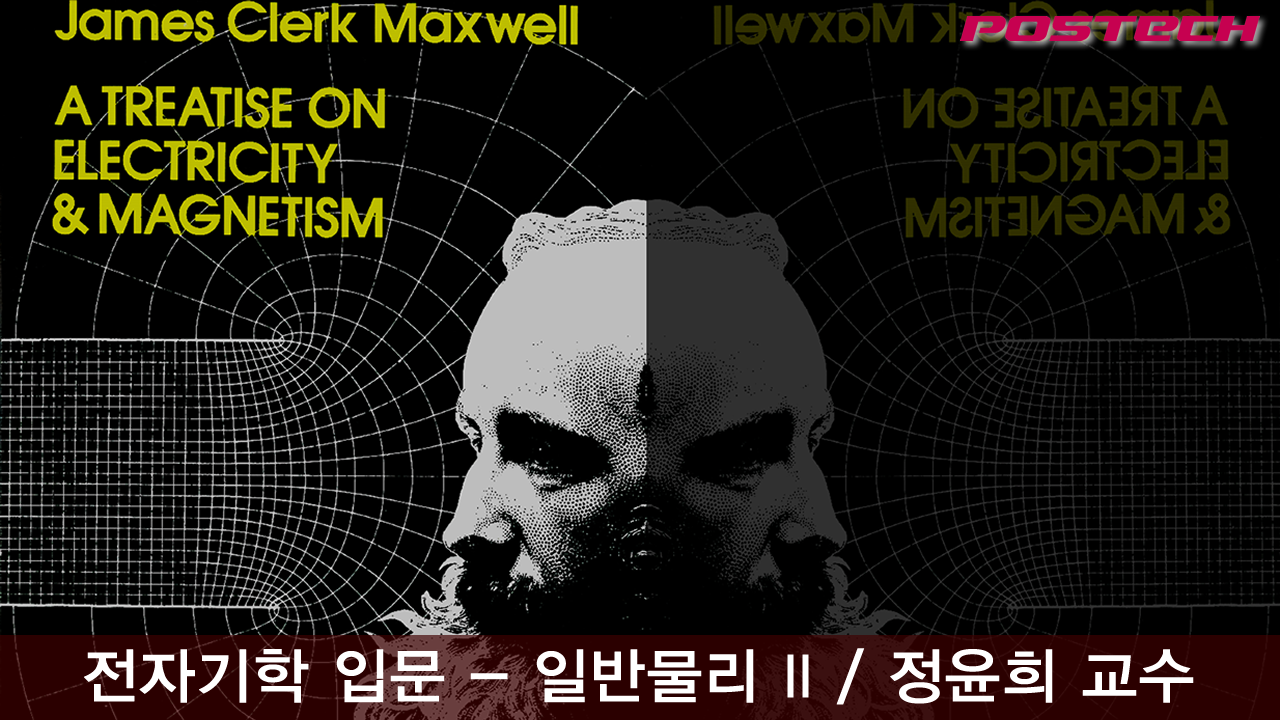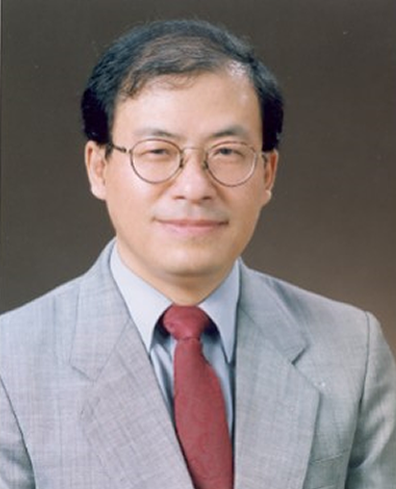## Course summary

• Type MOOC course
• Period Always open
• Learning Time Study freely
• Course approval method Automatic approval
• Certificate Issue Online
http://postech.edwith.org/electromagnetism
Thumb up 4 Learner 38### Instructor Introduction

•정윤희 교수

▣ 홈페이지: http://amos.postech.ac.kr/~yhj/yhj.html
▣ 주요 이력
▷ 시카고대학 물리학박사 (1987)
▷ 포스텍 교수 (1988 -)
▷ 물리학과 주임교수, 이학장 역임
▷ 스핀물성연구센터 소장 역임
▣ 주요 연구실적
▷ 양자기능성 물질 개발, 열물성 측정, 방사광 이용 물성 측정
▷ 논문 및 특허 다수
▣ 주요 수상경력
▷ 2011년 KRISS 측정과학상 수상
▷ 2011년 한국열물성학회 Netzsch award 수상
▷ 2004년 한국특허대전 국무총리상 수상
▷ 2001년 한국물리학회 학술상 수상

### Lecture plan

강의
1. CHAPTER 1
1. Overview
1. Problem Set 1-1
1. Electrostatics: Charge and Field
1. Problem Set 1-2
2. CHAPTER 2
1. Gauss’s Law 1
1. Problem Set 2-1
1. Gauss’s Law 2
1. Problem Set 2-2
3. CHAPTER 3
1. Electric Potential
1. Problem Set 3-1
1. Applications of Electric Potential
1. Problem Set 3-2
4. CHAPTER 4
1. Electrostatic Energy and Capacitors 1
1. Problem Set 4-1
1. Electrostatic Energy and Capacitors 2
1. Problem Set 4-2
5. CHAPTER 5
1. Current, Resistance, and EMF
1. Problem Set 5-1
1. Direct Current Circuits
1. Problem Set 5-2
6. CHAPTER 6
1. Magnetic Force and Magnetic Field
1. Problem Set 6-1
1. Magnetic Field and Motion of Charged Particles
1. Problem Set 6-2
7. CHAPTER 7
1. Sources of Magnetic Field - Electric Current
1. Problem Set 7-1
1. Sources of Magnetic Field - Magnetic Materials
1. Problem Set 7-2
8. CHAPTER 8
1. From statics to dynamics: Faraday induction
1. Problem Set 8-1
1. Induced electric field and its applications
1. Problem Set 8-2
9. CHAPTER 9
1. Inductors and magnetic energy
1. Problem Set 9-1
1. Alternating Current (AC) circuit
1. Problem Set 9-2
10. CHAPTER 10
1. Maxwell’s Equations
1. Problem Set 10-1
1. Electromagnetic waves
1. Problem Set 10-2
11. CHAPTER 11
1. EM radiation we call light
1. Problem Set 11-1
1. Dispersion and polarization of light
1. Problem Set 11-2
12. CHAPTER 12
1. Light reveals its wave nature 1 : interference
1. Problem Set 12-1
1. Light reveals its wave nature 2 : diffraction
1. Problem Set 12-2
13. CHAPTER 13
1. Maxwell's equations and principle of relativity
1. Problem Set 13-1
1. Special theory of relativity
1. Problem Set 13-2
14. Final Exam
1. Final Exam
1. Final Exam - 2